Скачать презентацию Introduction to CS 270 Math Foundations of CS

8c93366b4e6899ef622e49540b126d8f.ppt

• Количество слайдов: 32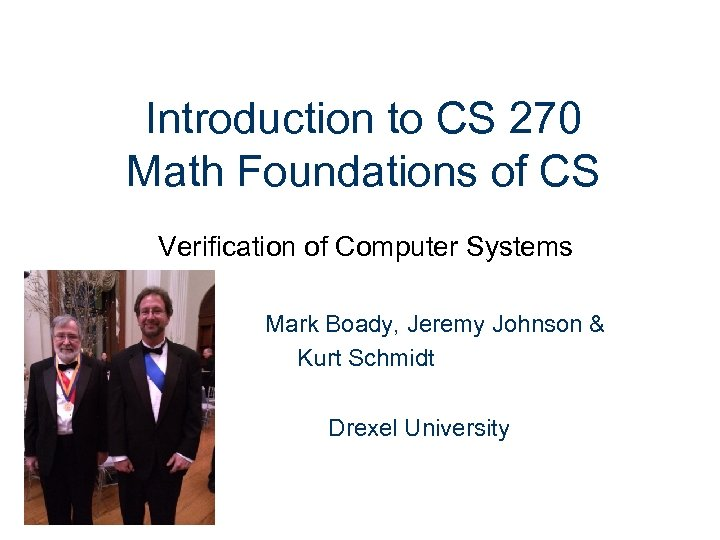Introduction to CS 270 Math Foundations of CS Verification of Computer Systems Mark Boady, Jeremy Johnson & Kurt Schmidt Drexel University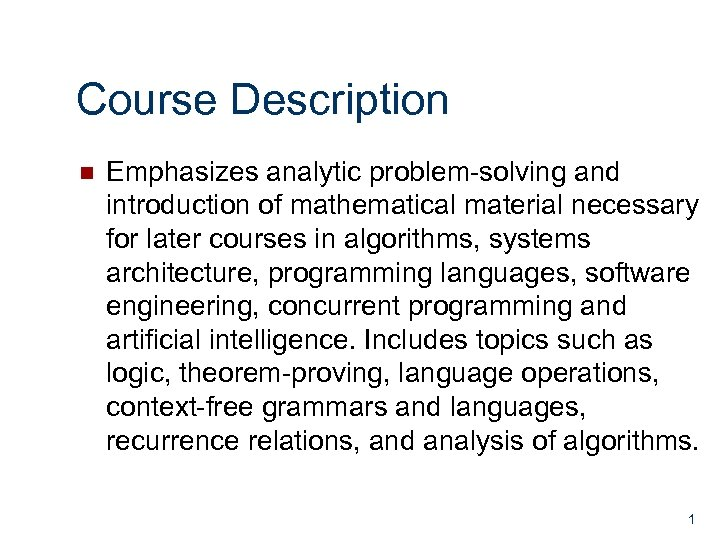Course Description n Emphasizes analytic problem-solving and introduction of mathematical material necessary for later courses in algorithms, systems architecture, programming languages, software engineering, concurrent programming and artificial intelligence. Includes topics such as logic, theorem-proving, language operations, context-free grammars and languages, recurrence relations, and analysis of algorithms. 1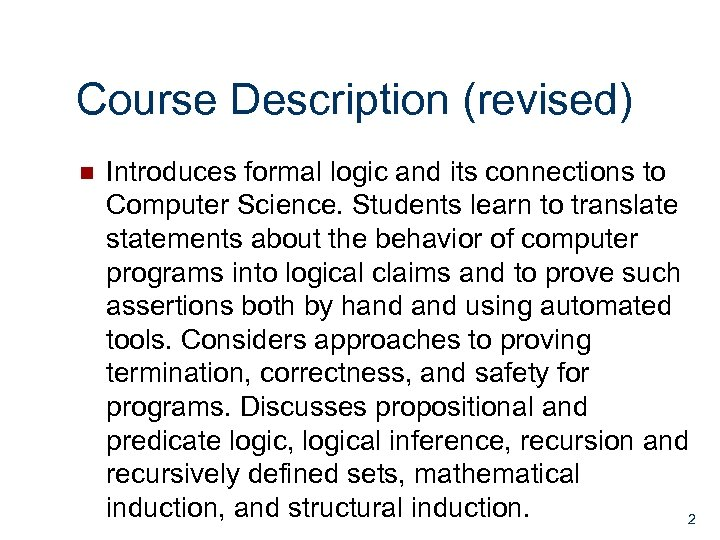Course Description (revised) n Introduces formal logic and its connections to Computer Science. Students learn to translate statements about the behavior of computer programs into logical claims and to prove such assertions both by hand using automated tools. Considers approaches to proving termination, correctness, and safety for programs. Discusses propositional and predicate logic, logical inference, recursion and recursively defined sets, mathematical induction, and structural induction. 2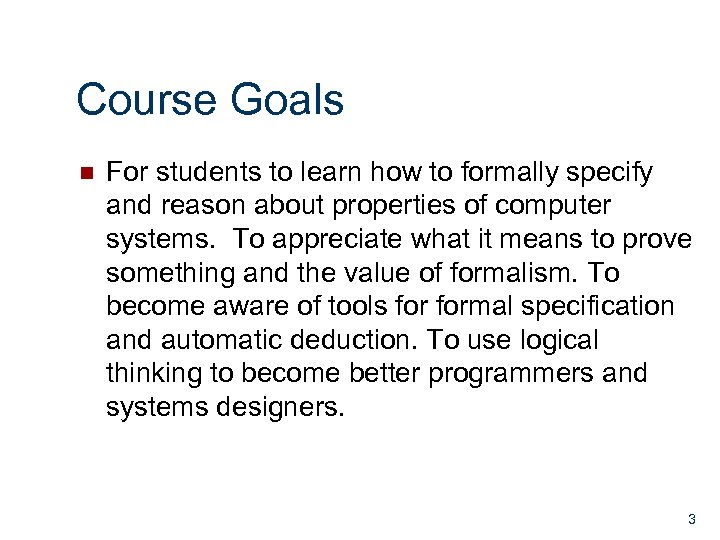Course Goals n For students to learn how to formally specify and reason about properties of computer systems. To appreciate what it means to prove something and the value of formalism. To become aware of tools formal specification and automatic deduction. To use logical thinking to become better programmers and systems designers. 3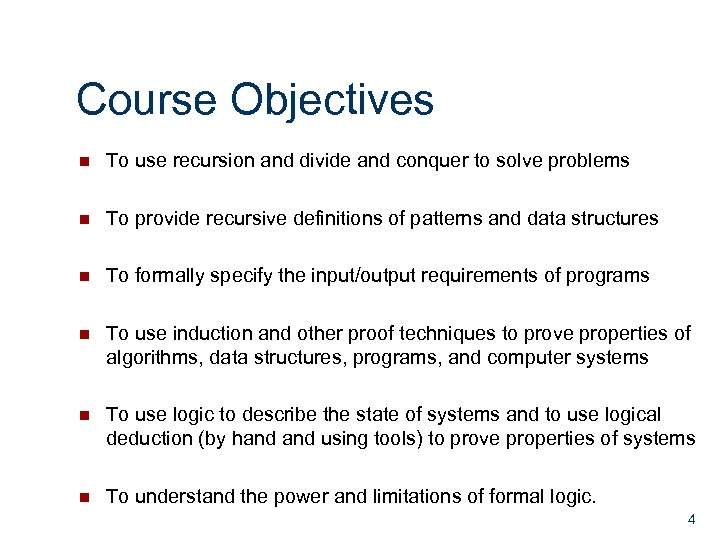Course Objectives n To use recursion and divide and conquer to solve problems n To provide recursive definitions of patterns and data structures n To formally specify the input/output requirements of programs n To use induction and other proof techniques to prove properties of algorithms, data structures, programs, and computer systems n To use logic to describe the state of systems and to use logical deduction (by hand using tools) to prove properties of systems n To understand the power and limitations of formal logic. 4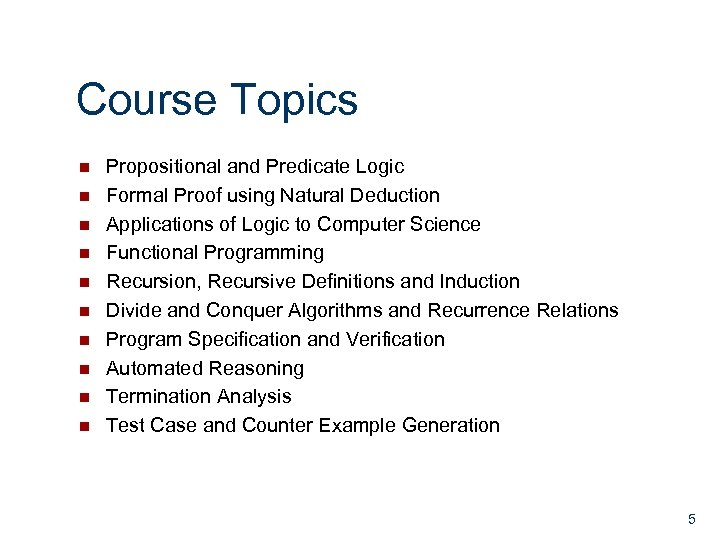Course Topics n n n n n Propositional and Predicate Logic Formal Proof using Natural Deduction Applications of Logic to Computer Science Functional Programming Recursion, Recursive Definitions and Induction Divide and Conquer Algorithms and Recurrence Relations Program Specification and Verification Automated Reasoning Termination Analysis Test Case and Counter Example Generation 5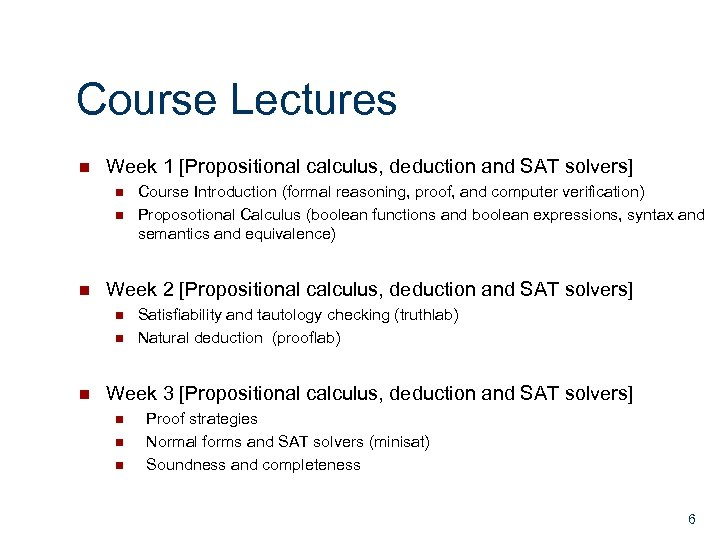Course Lectures n Week 1 [Propositional calculus, deduction and SAT solvers] n n n Week 2 [Propositional calculus, deduction and SAT solvers] n n n Course Introduction (formal reasoning, proof, and computer verification) Proposotional Calculus (boolean functions and boolean expressions, syntax and semantics and equivalence) Satisfiability and tautology checking (truthlab) Natural deduction (prooflab) Week 3 [Propositional calculus, deduction and SAT solvers] n n n Proof strategies Normal forms and SAT solvers (minisat) Soundness and completeness 6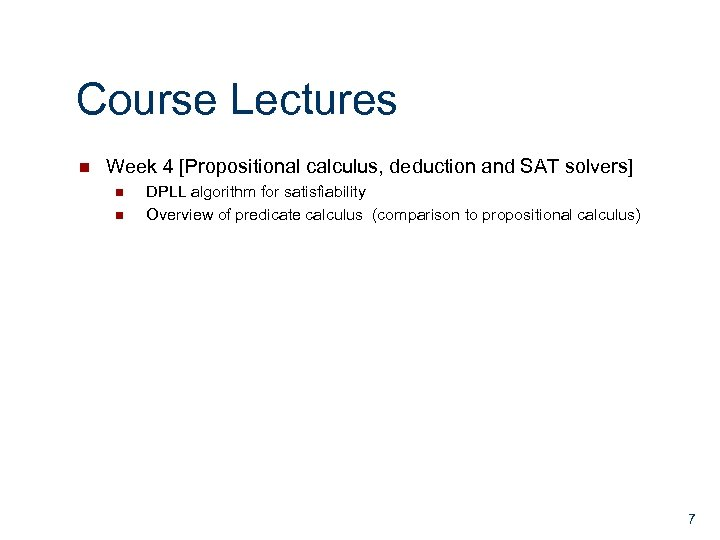Course Lectures n Week 4 [Propositional calculus, deduction and SAT solvers] n n DPLL algorithm for satisfiability Overview of predicate calculus (comparison to propositional calculus) 7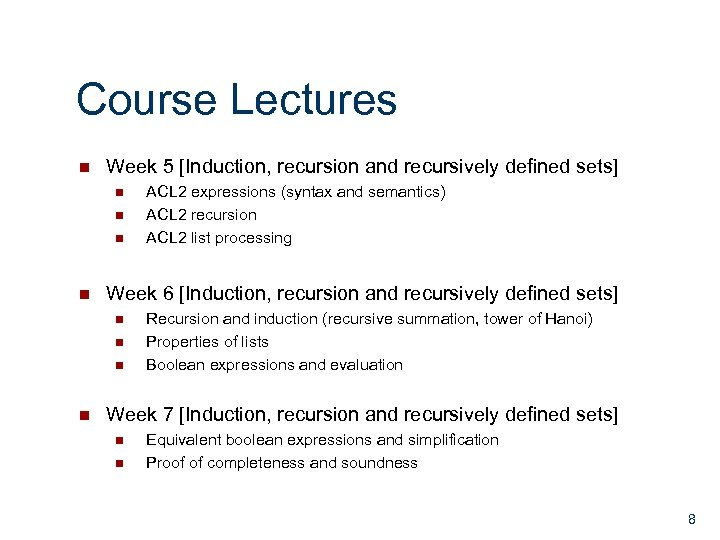Course Lectures n Week 5 [Induction, recursion and recursively defined sets] n n Week 6 [Induction, recursion and recursively defined sets] n n ACL 2 expressions (syntax and semantics) ACL 2 recursion ACL 2 list processing Recursion and induction (recursive summation, tower of Hanoi) Properties of lists Boolean expressions and evaluation Week 7 [Induction, recursion and recursively defined sets] n n Equivalent boolean expressions and simplification Proof of completeness and soundness 8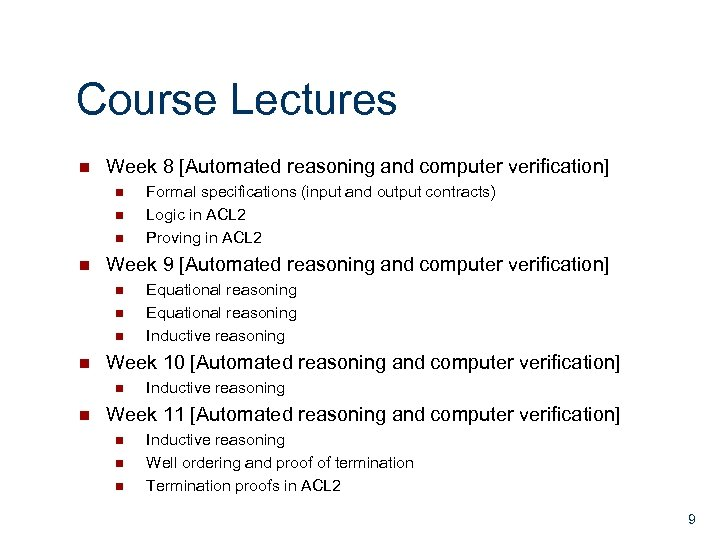Course Lectures n Week 8 [Automated reasoning and computer verification] n n Week 9 [Automated reasoning and computer verification] n n Equational reasoning Inductive reasoning Week 10 [Automated reasoning and computer verification] n n Formal specifications (input and output contracts) Logic in ACL 2 Proving in ACL 2 Inductive reasoning Week 11 [Automated reasoning and computer verification] n n n Inductive reasoning Well ordering and proof of termination Termination proofs in ACL 2 9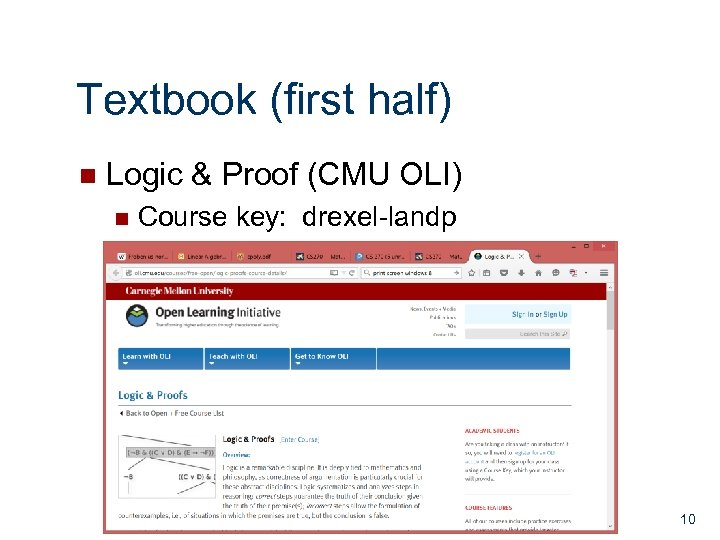Textbook (first half) n Logic & Proof (CMU OLI) n Course key: drexel-landp 10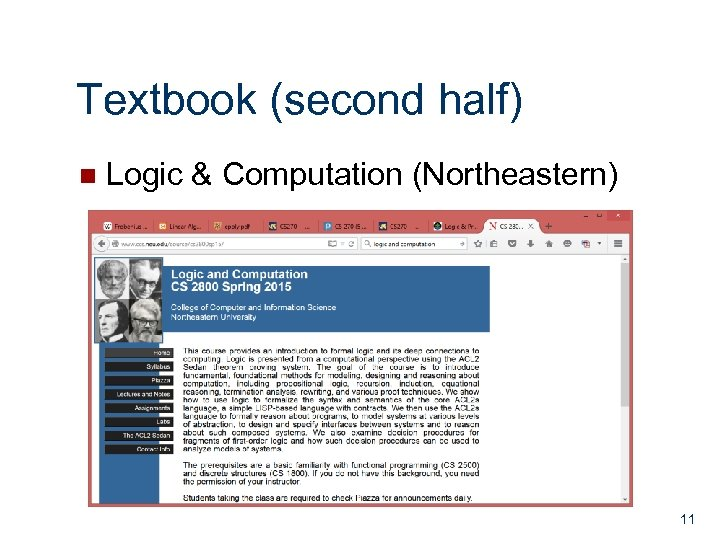Textbook (second half) n Logic & Computation (Northeastern) 11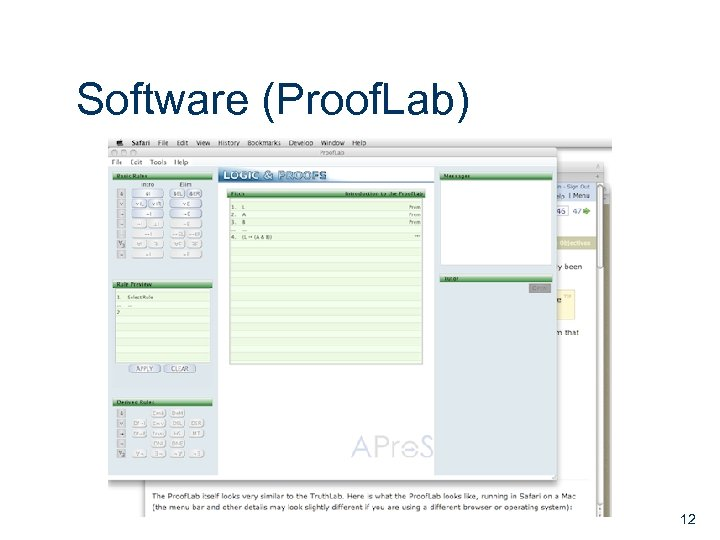Software (Proof. Lab) 12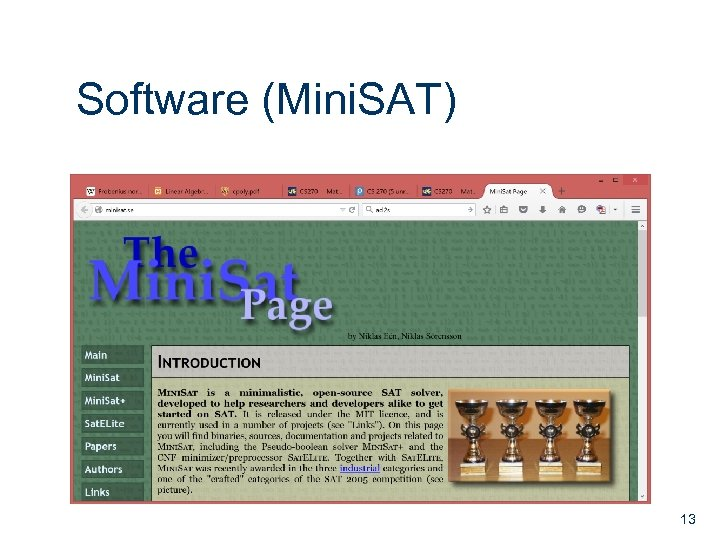Software (Mini. SAT) 13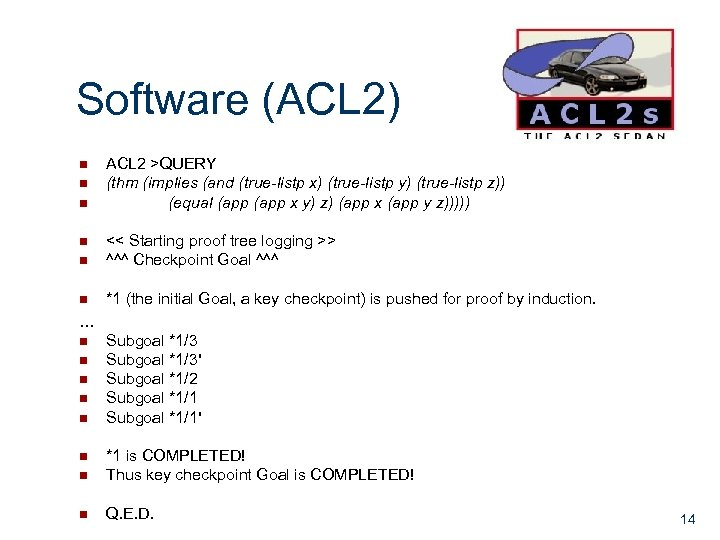Software (ACL 2) n n n ACL 2 >QUERY (thm (implies (and (true-listp x) (true-listp y) (true-listp z)) (equal (app x y) z) (app x (app y z))))) n << Starting proof tree logging >> ^^^ Checkpoint Goal ^^^ n *1 (the initial Goal, a key checkpoint) is pushed for proof by induction. n … n n n Subgoal *1/3' Subgoal *1/2 Subgoal *1/1' n *1 is COMPLETED! Thus key checkpoint Goal is COMPLETED! n Q. E. D. n 14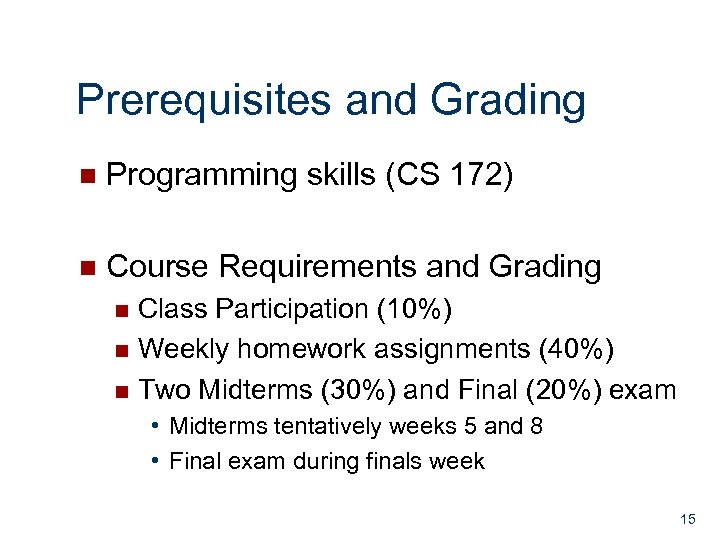Prerequisites and Grading n Programming skills (CS 172) n Course Requirements and Grading Class Participation (10%) n Weekly homework assignments (40%) n Two Midterms (30%) and Final (20%) exam n • Midterms tentatively weeks 5 and 8 • Final exam during finals week 15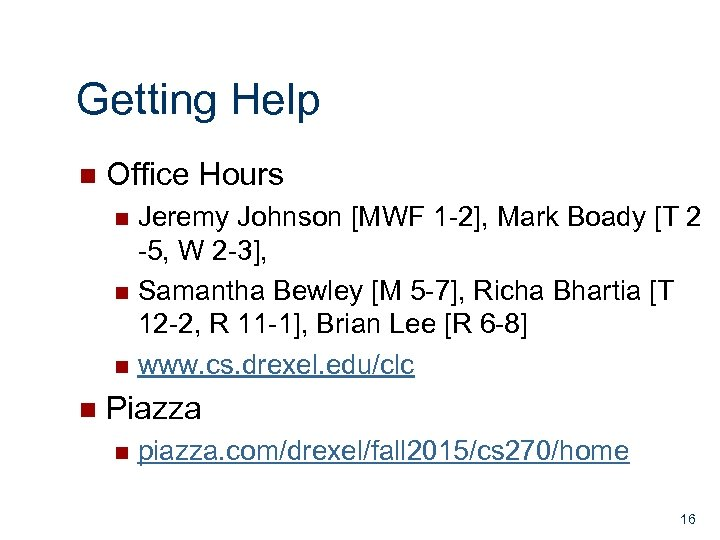Getting Help n Office Hours Jeremy Johnson [MWF 1 -2], Mark Boady [T 2 -5, W 2 -3], n Samantha Bewley [M 5 -7], Richa Bhartia [T 12 -2, R 11 -1], Brian Lee [R 6 -8] n www. cs. drexel. edu/clc n n Piazza n piazza. com/drexel/fall 2015/cs 270/home 16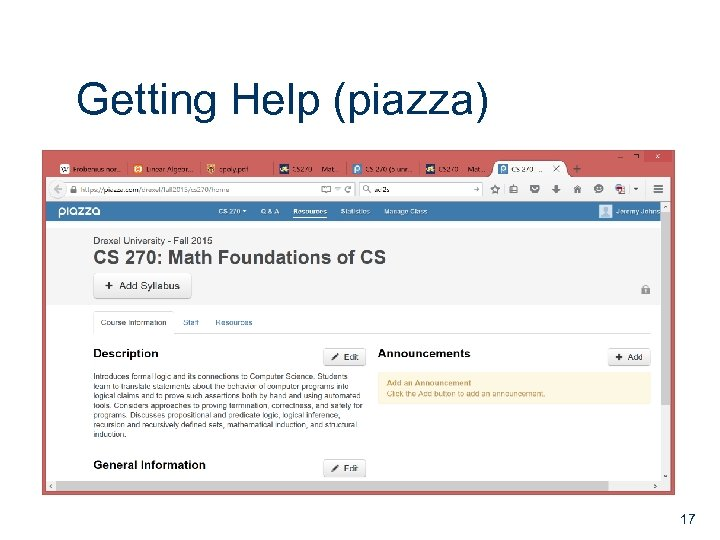Getting Help (piazza) 17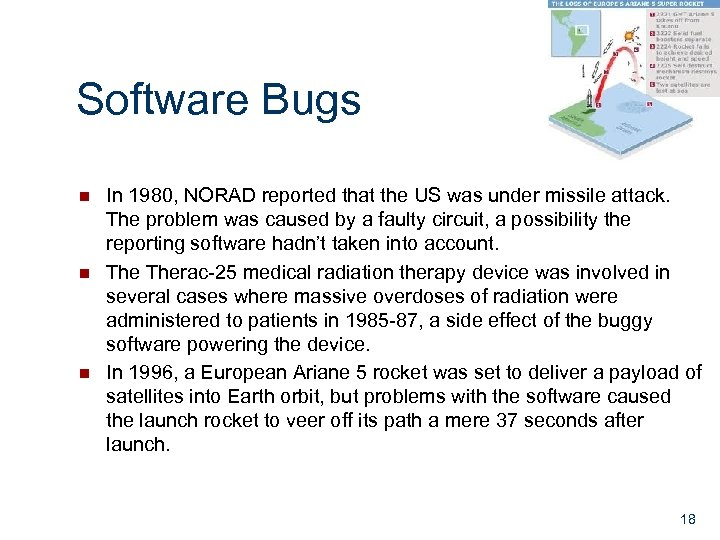Software Bugs n n n In 1980, NORAD reported that the US was under missile attack. The problem was caused by a faulty circuit, a possibility the reporting software hadn’t taken into account. Therac-25 medical radiation therapy device was involved in several cases where massive overdoses of radiation were administered to patients in 1985 -87, a side effect of the buggy software powering the device. In 1996, a European Ariane 5 rocket was set to deliver a payload of satellites into Earth orbit, but problems with the software caused the launch rocket to veer off its path a mere 37 seconds after launch. 18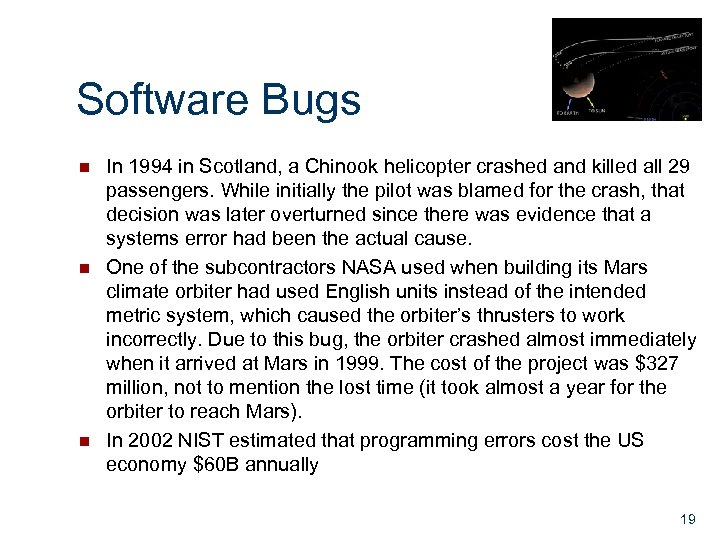Software Bugs n n n In 1994 in Scotland, a Chinook helicopter crashed and killed all 29 passengers. While initially the pilot was blamed for the crash, that decision was later overturned since there was evidence that a systems error had been the actual cause. One of the subcontractors NASA used when building its Mars climate orbiter had used English units instead of the intended metric system, which caused the orbiter’s thrusters to work incorrectly. Due to this bug, the orbiter crashed almost immediately when it arrived at Mars in 1999. The cost of the project was \$327 million, not to mention the lost time (it took almost a year for the orbiter to reach Mars). In 2002 NIST estimated that programming errors cost the US economy \$60 B annually 19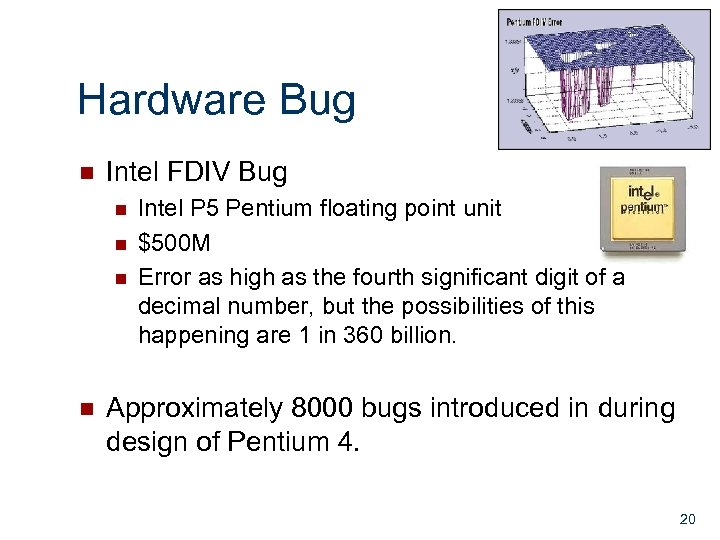Hardware Bug n Intel FDIV Bug n n Intel P 5 Pentium floating point unit \$500 M Error as high as the fourth significant digit of a decimal number, but the possibilities of this happening are 1 in 360 billion. Approximately 8000 bugs introduced in during design of Pentium 4. 20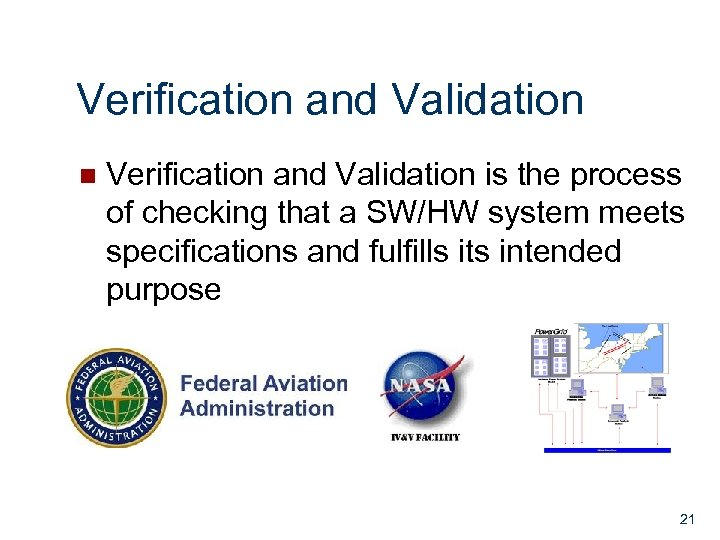Verification and Validation n Verification and Validation is the process of checking that a SW/HW system meets specifications and fulfills its intended purpose 21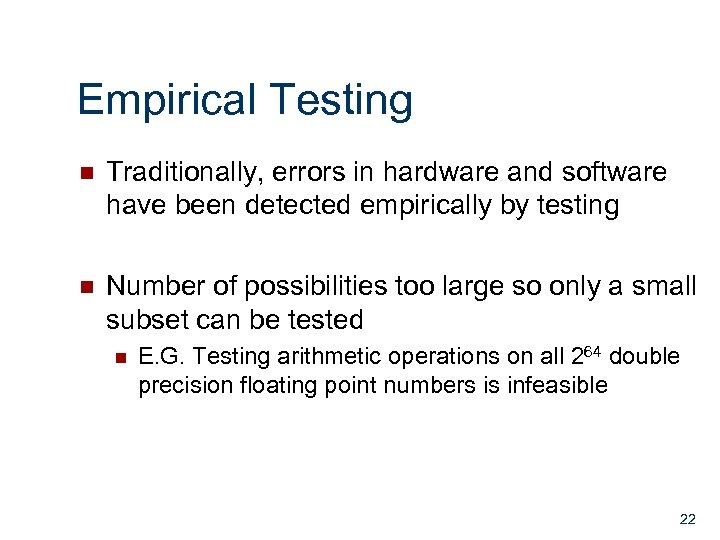Empirical Testing n Traditionally, errors in hardware and software have been detected empirically by testing n Number of possibilities too large so only a small subset can be tested n E. G. Testing arithmetic operations on all 264 double precision floating point numbers is infeasible 22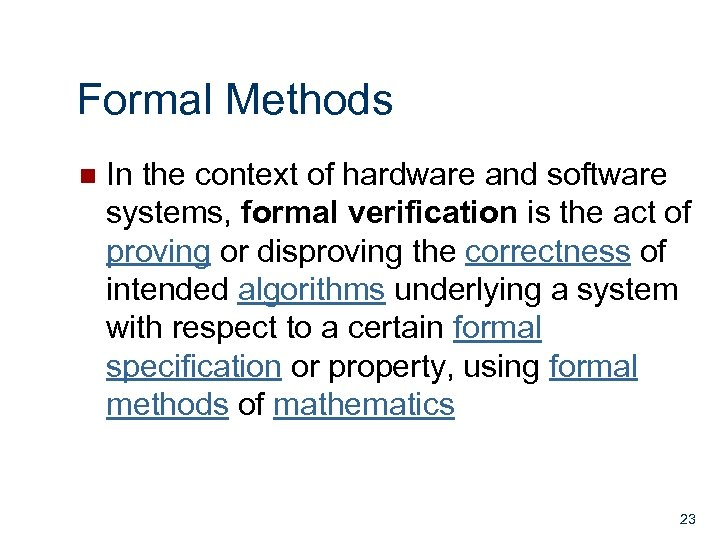Formal Methods n In the context of hardware and software systems, formal verification is the act of proving or disproving the correctness of intended algorithms underlying a system with respect to a certain formal specification or property, using formal methods of mathematics 23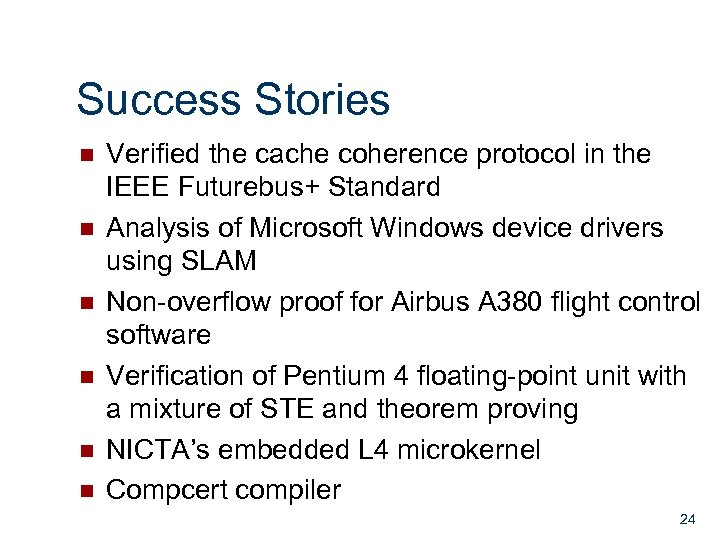Success Stories n n n Verified the cache coherence protocol in the IEEE Futurebus+ Standard Analysis of Microsoft Windows device drivers using SLAM Non-overflow proof for Airbus A 380 flight control software Verification of Pentium 4 floating-point unit with a mixture of STE and theorem proving NICTA’s embedded L 4 microkernel Compcert compiler 24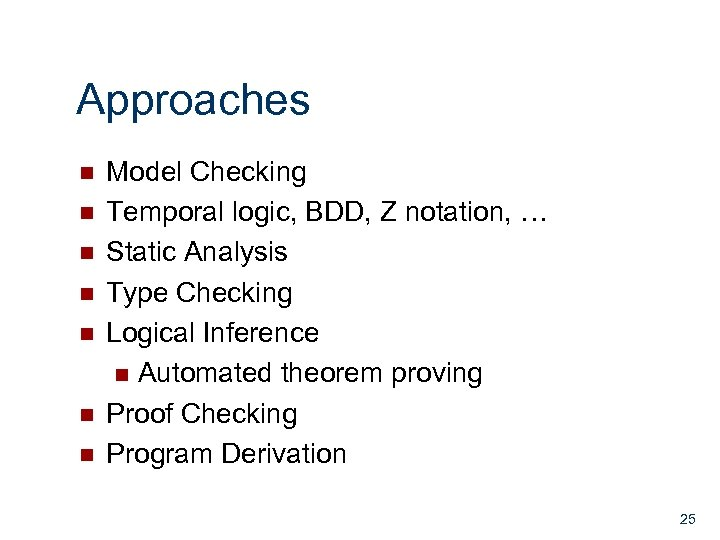Approaches n n n n Model Checking Temporal logic, BDD, Z notation, … Static Analysis Type Checking Logical Inference n Automated theorem proving Proof Checking Program Derivation 25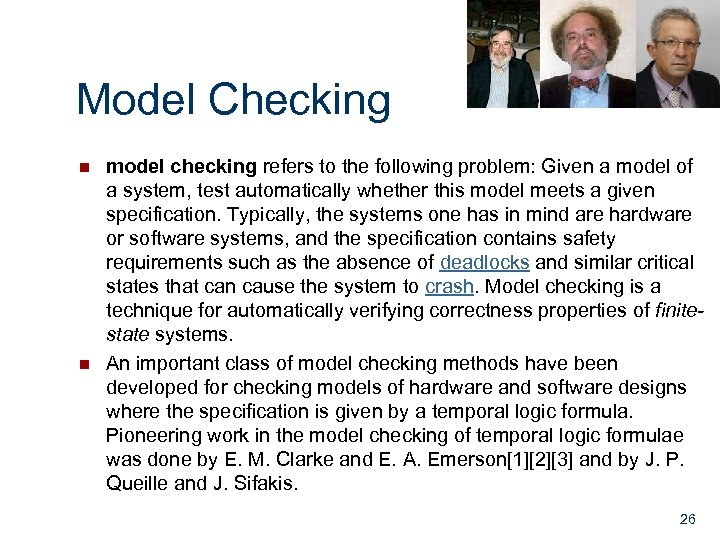Model Checking n n model checking refers to the following problem: Given a model of a system, test automatically whether this model meets a given specification. Typically, the systems one has in mind are hardware or software systems, and the specification contains safety requirements such as the absence of deadlocks and similar critical states that can cause the system to crash. Model checking is a technique for automatically verifying correctness properties of finitestate systems. An important class of model checking methods have been developed for checking models of hardware and software designs where the specification is given by a temporal logic formula. Pioneering work in the model checking of temporal logic formulae was done by E. M. Clarke and E. A. Emerson and by J. P. Queille and J. Sifakis. 26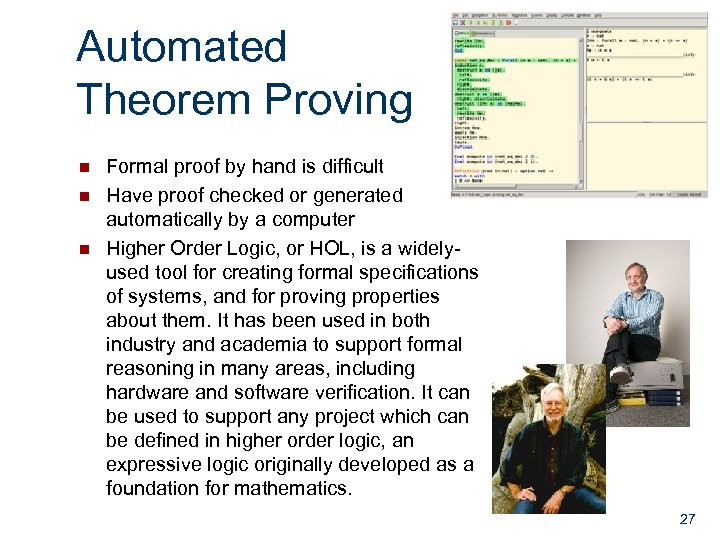Automated Theorem Proving n n n Formal proof by hand is difficult Have proof checked or generated automatically by a computer Higher Order Logic, or HOL, is a widelyused tool for creating formal specifications of systems, and for proving properties about them. It has been used in both industry and academia to support formal reasoning in many areas, including hardware and software verification. It can be used to support any project which can be defined in higher order logic, an expressive logic originally developed as a foundation for mathematics. 27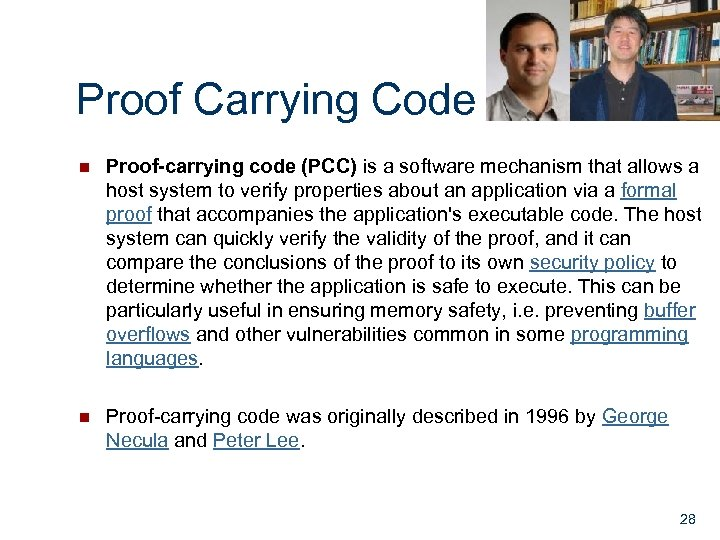Proof Carrying Code n Proof-carrying code (PCC) is a software mechanism that allows a host system to verify properties about an application via a formal proof that accompanies the application's executable code. The host system can quickly verify the validity of the proof, and it can compare the conclusions of the proof to its own security policy to determine whether the application is safe to execute. This can be particularly useful in ensuring memory safety, i. e. preventing buffer overflows and other vulnerabilities common in some programming languages. n Proof-carrying code was originally described in 1996 by George Necula and Peter Lee. 28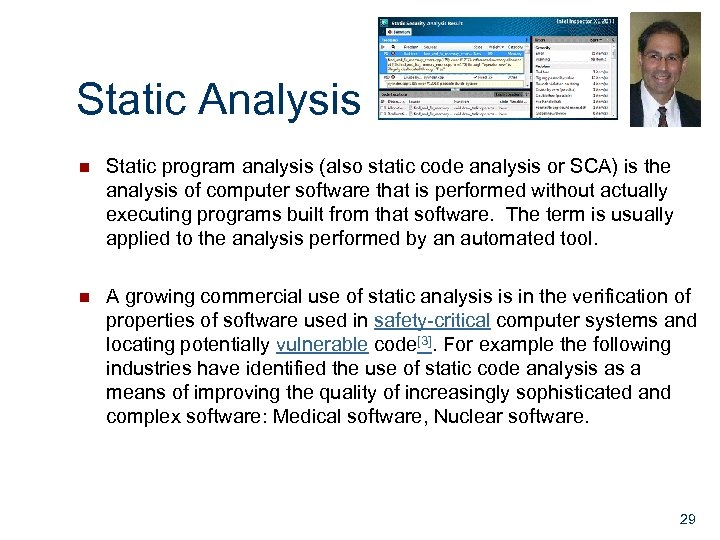Static Analysis n Static program analysis (also static code analysis or SCA) is the analysis of computer software that is performed without actually executing programs built from that software. The term is usually applied to the analysis performed by an automated tool. n A growing commercial use of static analysis is in the verification of properties of software used in safety-critical computer systems and locating potentially vulnerable code. For example the following industries have identified the use of static code analysis as a means of improving the quality of increasingly sophisticated and complex software: Medical software, Nuclear software. 29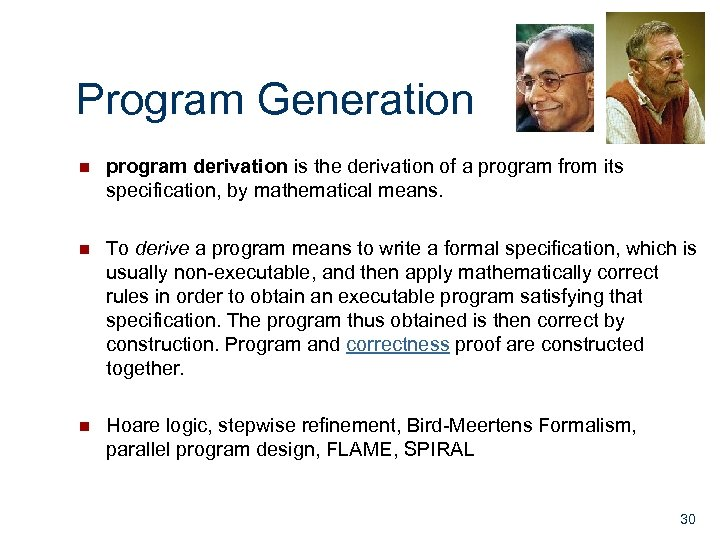Program Generation n program derivation is the derivation of a program from its specification, by mathematical means. n To derive a program means to write a formal specification, which is usually non-executable, and then apply mathematically correct rules in order to obtain an executable program satisfying that specification. The program thus obtained is then correct by construction. Program and correctness proof are constructed together. n Hoare logic, stepwise refinement, Bird-Meertens Formalism, parallel program design, FLAME, SPIRAL 30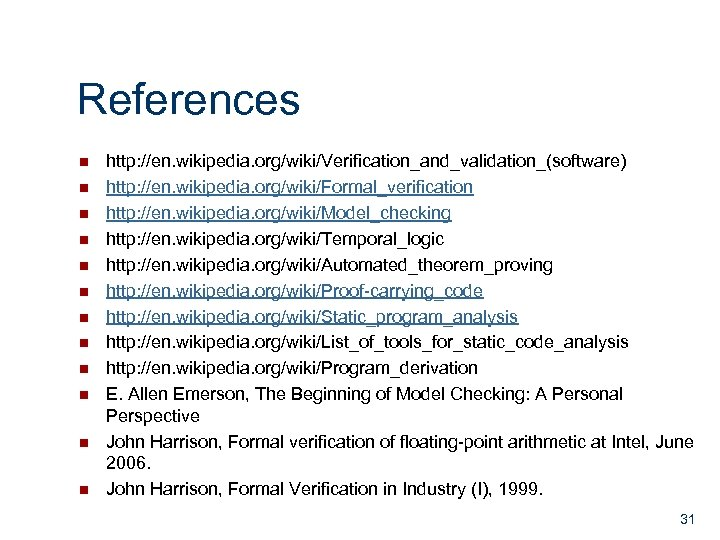References n n n http: //en. wikipedia. org/wiki/Verification_and_validation_(software) http: //en. wikipedia. org/wiki/Formal_verification http: //en. wikipedia. org/wiki/Model_checking http: //en. wikipedia. org/wiki/Temporal_logic http: //en. wikipedia. org/wiki/Automated_theorem_proving http: //en. wikipedia. org/wiki/Proof-carrying_code http: //en. wikipedia. org/wiki/Static_program_analysis http: //en. wikipedia. org/wiki/List_of_tools_for_static_code_analysis http: //en. wikipedia. org/wiki/Program_derivation E. Allen Emerson, The Beginning of Model Checking: A Personal Perspective John Harrison, Formal verification of floating-point arithmetic at Intel, June 2006. John Harrison, Formal Verification in Industry (I), 1999. 31